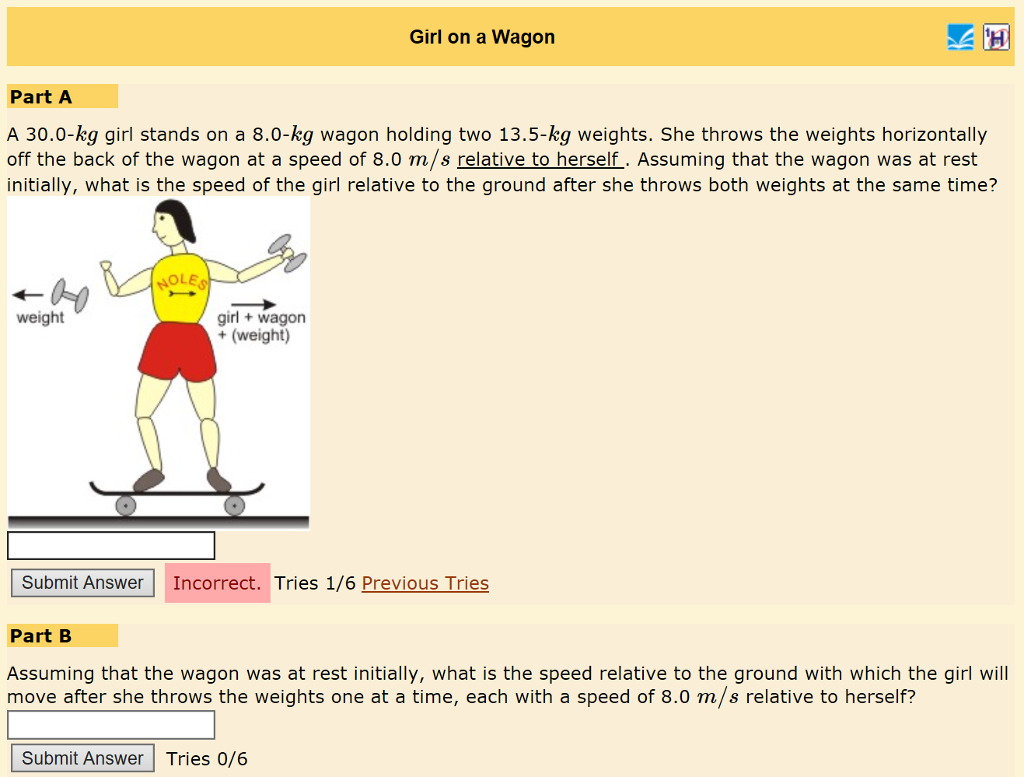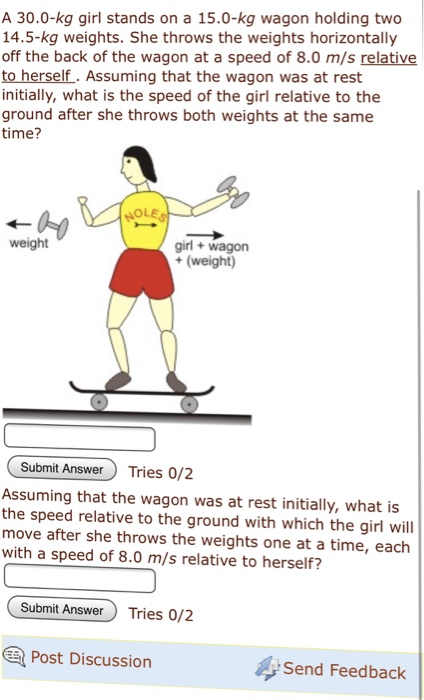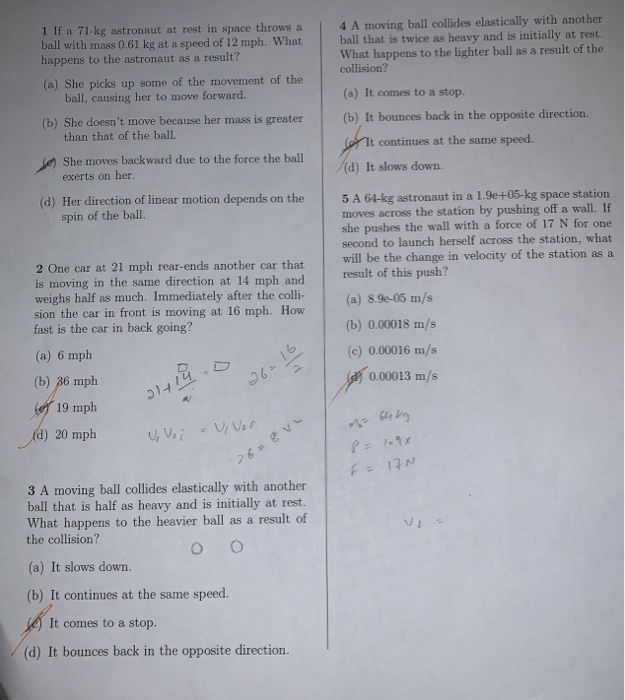# Buffy is rolling along in her 13.0 kg wagon at 3.0 m/s (in the positive direction)...

Buffy is rolling along in her 13.0 kg wagon at 3.0 m/s (in the positive direction) when she jumps off the back. She continues to move forward at 1.5 m/s relative to the ground. This causes her wagon to go speeding forward at 7.00 m/s relative to the ground. How much does Buffy weigh?

let mass of buffy be M.

then using conservation of linear momentum:

(13+M)*3=M*1.5+13*7

M=34.667 kg

SOLUTION :

Let Buffy weighs W kg .

So, momentum of both Buffy and wagon before separation

= (W + 13) * 3

= 3 W + 39   kg-m/s

Momentum after Buffy separates from the wagon

= W * 1.5 + 13 * 7

= 1.5 W + 91 kg-m/sec

Momentum remains unchanged, so,

3 W + 39 = 1.5 W + 91

=> (3 - 1.5) W = 91 - 39

=> 1.5 W = 52

=> W = 52/1.5

=> W = 34.67 kg

So, weight of Buffy is 34.67 kg (ANSWER).

#### Earn Coin

Coins can be redeemed for fabulous gifts.

Similar Homework Help Questions
• ### Girl on a Wagon Part A A 30.0-kg girl stands on a 8.0-kg wagon holding two...Girl on a Wagon Part A A 30.0-kg girl stands on a 8.0-kg wagon holding two 13.5-kg weights. She throws the weights horizontally off the back of the wagon at a speed of 8.0 m/s relative to herself_. Assuming that the wagon was at rest initially, what is the speed of the girl relative to the ground after she throws both weights at the same time? NOLE weight girl +wagon +(weight) Submit Answer Incorrect. Tries 1/6 Previous Tries Part B...

• ### 1. A 45 kg girl stands on a 7 kg wagon holding two 21 kg weights....

1. A 45 kg girl stands on a 7 kg wagon holding two 21 kg weights. She throws the weights horizontally off the back of the wagon at a speed of 8 m/s relative to herself. a)Assuming that the wagon was at rest initially, what's the speed of the girl relative to the ground after she throws both weights at the same time? b)If the wagon was initially at rest, what's the speed relative to the ground with which the...

• ### A 35.0-kg girl stands on a 5.0-kg wagon holding two 17.5-kg weights. She throws the weights...

A 35.0-kg girl stands on a 5.0-kg wagon holding two 17.5-kg weights. She throws the weights horizontally off the back of the wagon at a speed of 3.5 m/s relative to herself . Assuming that the wagon was at rest initially, what is the speed of the girl relative to the ground after she throws both weights at the same time? in m/s Assuming that the wagon was at rest initially, what is the speed relative to the ground with...

• ### A 30.0-kg girl stands on a 15.0-kg wagon holding two 14.5-kg weights. She throws the weights...A 30.0-kg girl stands on a 15.0-kg wagon holding two 14.5-kg weights. She throws the weights horizontally off the back of the wagon at a speed of 8.0 m/s relative to herself. Assuming that the wagon was at rest initially, what is the speed of the girl relative to the ground after she throws both weights at the same time? girl+ wagon (weight weight Submit Answer Tries 0/2 Assuming that the wagon was at rest initially, what is the speed...

• ### answer all please 1 If a 71-kg astronaut at rest in space throws a ball with...answer all please 1 If a 71-kg astronaut at rest in space throws a ball with mass 0.61 kg at a speed of 12 mph. What happens to the astronaut as a result? 4 A moving ball collides elastically with another ball that is twice as heavy and is initially at rest. What happens to the lighter ball as a result of the collision? (a) She picks up some of the movement of the ball, causing her to move forward....

• ### a 2.0 kg mass moves along a frictionless horizontal surface at a speed of 5.0 m/s....

a 2.0 kg mass moves along a frictionless horizontal surface at a speed of 5.0 m/s. The mass encounters a 30 degree inclined surface with a constant friction force of 1.5 N. At 1 m high (vertical) the surface levels off and is again frictionless. the mass then encounters a spring with k=10 N/m a) how far is the spring compressed after the mass comes to rest? b) how far down the inclined plane will the mass move after bouncing...

Please read the article and answer about questions. You and the Law Business and law are inseparable. For B-Money, the two predictably merged when he was negotiat- ing a deal for his tracks. At other times, the merger is unpredictable, like when your business faces an unexpected auto accident, product recall, or government regulation change. In either type of situation, when business owners know the law, they can better protect themselves and sometimes even avoid the problems completely. This chapter...